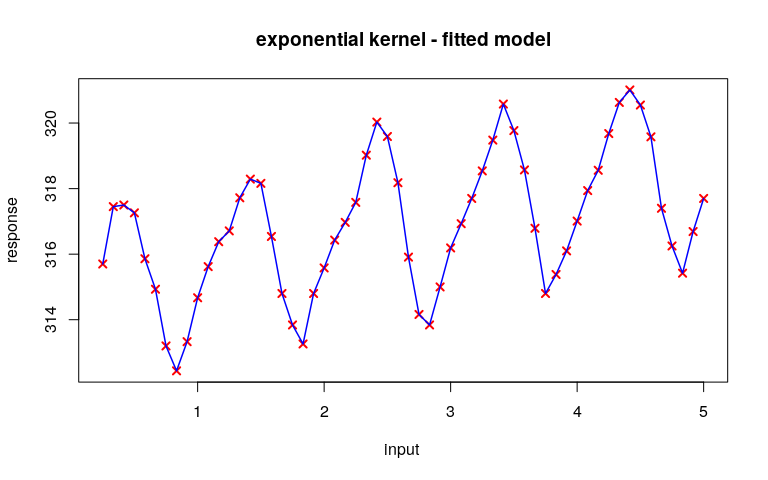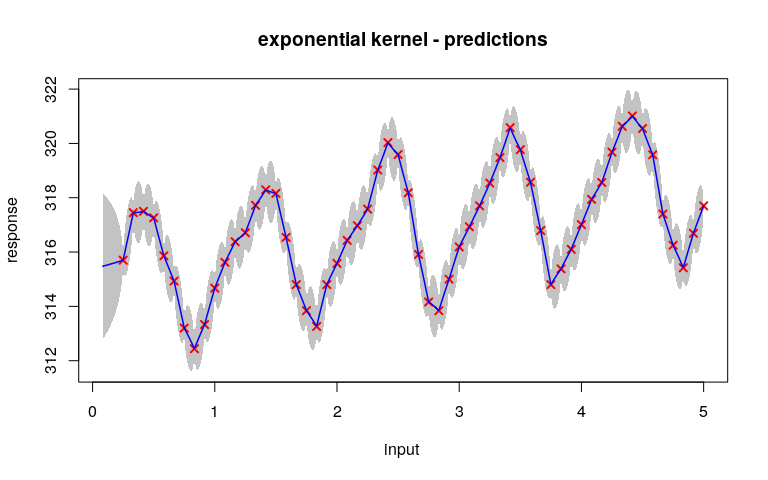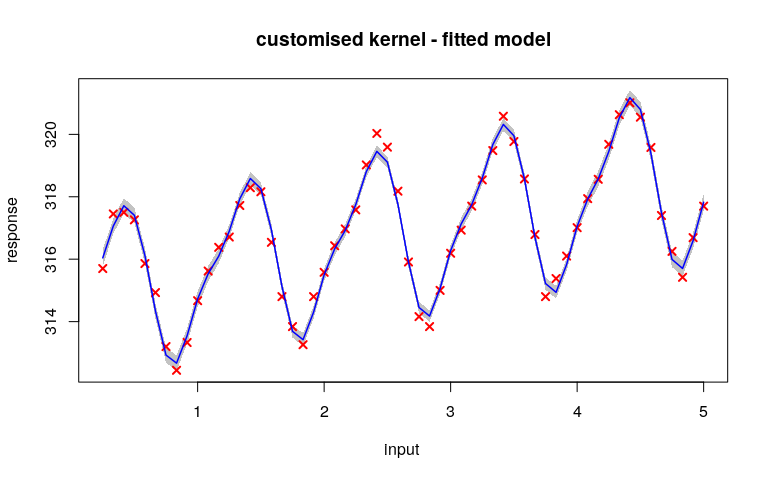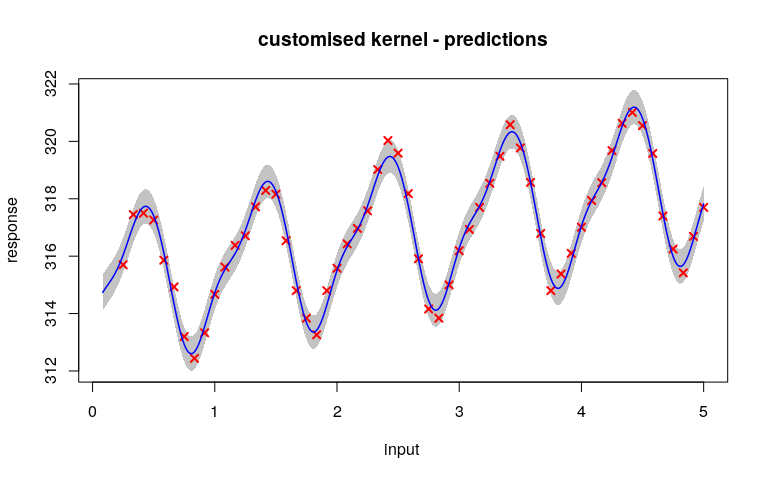# co2 data example

In this example, we use a real dataset and apply a customised covariance kernel. See the documentation of the package for a description of this dataset.

library(GPFDA)
data(co2, package = "GPFDA")

For visualisation purposes, we will use only the first five years of the sample.

y <- c(t(data.matrix(co2[1:5, 1:12])))
n <- length(y)
input <- 5*(1:n)/n
whichNeg <- (y<0) # removing negative values
y <- y[!whichNeg]
input <- input[!whichNeg]

We want to fit a GPR model using the observed data and then make predictions at a $$1000$$ equally spaced time points:

inputNew <- 5*seq(1/n, 1, length.out=1000) # input test for prediction

## Using exponential covariance kernel

We will first fit a GPR model with a linear trend line for the mean function by setting meanModel='t', and a exponential covariance function by setting Cov='pow.ex' and gamma=1.

set.seed(789)
fit1 <- gpr(input=input, response=y, Cov='pow.ex', gamma=1, meanModel='t',
nInitCandidates=50, trace=2)
sapply(fit1$hyper, exp) #> pow.ex.v pow.ex.w vv #> 3.330e+00 2.483e+00 3.162e-09 Since the noise variance vv estimate is extremely low, this fitted model basically interpolates the datapoints: plot(fit1, main="exponential kernel - fitted model")See below the large uncertainty in predictions for test input points: pred1 <- gprPredict(train = fit1, inputNew=inputNew) plot(pred1, main="exponential kernel - predictions")These results suggest that the exponential kernel is likely not to be the best choice. A kernel which takes into account a periodic pattern may be wanted. ## Using a customised covariance kernel To capture the periodic pattern, we use the following covariance function: $\begin{equation} k(t,t')=v \exp(-w (t-t')^2 - u \sin^2(\pi (t-t'))), \quad v,w,u > 0 \label{cus-cov} \end{equation}$ (see Williams, C. K., & Rasmussen, C. E. (2006). “Gaussian Processes for Machine Learning”, the MIT press). This is not a standard covariance kernel and is not included in the package. Therefore, we will define it manually. ### Defining a customised covariance kernel The first step is to define the covariance kernel itself. The name of the kernel must be constituted by the prefix cov. and 6 letters ( e.g., cov.custom). Here the C++ function distMat is used to calculate distances $$\exp(w)(t-t')^2$$. The similar distMatSq function is used when t and t' are identical. See package documentation for more details. cov.custom <- function(hyper, input, inputNew=NULL){ hyper <- lapply(hyper, exp) if(is.null(inputNew)){ inputNew <- as.matrix(input) }else{ inputNew <- as.matrix(inputNew) } input <- as.matrix(input) A1 <- distMat(input=input, inputNew=inputNew, A=as.matrix(hyper$custom.w),
power=2)
sept <- outer(input[,1], inputNew[,1], "-")
A2 <- hyper$custom.u*(sin(pi*sept))^2 customCov <- hyper$custom.v*exp(-A1-A2)
return(customCov)
}

### Defining the first derivatives

The second step is to define the first derivative of the log-likelihood with respect to each of the hyperparameters of the covariance kernel.

The name of the functions should look like Dloglik.custom.w, where the middle affix is the custom defined name, and the last letter (i.e. w) names the vector of the hyperparameters.

For details about derivatives, see Shi, J. Q., and Choi, T. (2011), “Gaussian Process Regression Analysis for Functional Data”, CRC Press.

Dloglik.custom.w <- function(hyper, input, AlphaQ){
A1 <- distMatSq(input=input, A=as.matrix(exp(hyper$custom.w)), power=2) Dcov <- - cov.custom(hyper, input)*A1 out <- 0.5*sum(diag(AlphaQ%*%Dcov)) return(out) } Dloglik.custom.u <- function(hyper, input, AlphaQ){ sept <- outer(input[,1], input[,1], "-") A2 <- exp(hyper$custom.u)*(sin(pi*sept))^2
Dcov <- - cov.custom(hyper, input)*A2
out <- 0.5*sum(diag(AlphaQ%*%Dcov))
return(out)
}

Dloglik.custom.v <- function(hyper, input, AlphaQ){
Dcov <- cov.custom(hyper, input)
out <- 0.5*sum(diag(AlphaQ%*%Dcov))
return(out)
}

### Defining the second derivatives

The third step is to define the second derivatives of the log-likelihood.

D2custom.w <- function(hyper, input, inv.Q, Alpha.Q){
Cov <- cov.custom(hyper, input)
A1 <- distMatSq(input=input, A=as.matrix(exp(hyper$custom.w)), power=2) D1cov <- -Cov*A1 D2cov <- -(D1cov + Cov)*A1 D2c.w <- D2(D1cov, D2cov, inv.Q, Alpha.Q) return(D2c.w) } D2custom.u <- function(hyper, input, inv.Q, Alpha.Q){ Cov <- cov.custom(hyper, input) sept <- outer(input[,1], input[,1], "-") A2 <- exp(hyper$custom.u)*(sin(pi*sept))^2
D1cov <- - Cov*A2
D2cov <- -(D1cov + Cov)*A2
D2c.u <- D2(D1cov, D2cov, inv.Q, Alpha.Q)
return(D2c.u)
}

D2custom.v <- function(hyper, input, inv.Q, Alpha.Q){
out <- cov.custom(hyper, input)
return(out)
}

### Defining a diagonal matrix including the signal variance

Finally, we define a function to calculate a diagonal matrix contanining the variance of the customised covariance function. This is used for making predictions at new input points.

diag.custom <- function(hyper, input){
Qstar <- rep(exp(hyper$custom.v), nrow(input)) return(Qstar) } ### Fitting the GPR model with the customised kernel and making predictions fit2 <- gpr(input=input, response=y, Cov='custom', NewHyper=c('custom.w','custom.u','custom.v'), gamma=2, meanModel='t', nInitCandidates=50, trace=2, useGradient = F) sapply(fit2$hyper, exp)
#>   custom.u   custom.v   custom.w         vv
#>  0.4885247 12.5995047  0.0007988  0.0779593

Note that now the noise variance vv estimate is no longer so small. Both the fitted model and the predictions seem much more reasonable for this dataset:

plot(fit2, main="customised kernel - fitted model")#> Predicted values not found, ploting fitted values
pred2 <- gprPredict(train = fit2, inputNew=inputNew)
plot(pred2, main="customised kernel - predictions")